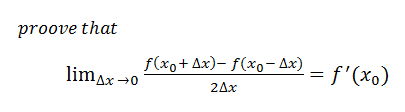# limits of different functions

## Recommended Posts

I am currently reading a book on calculus and I have come across a problem which I can't solve. I do feel like the answer is something simple. Please note that I am fairly new to calculus.Thank you.

##### Share on other sites

What is your definition of f'(x) ?

Are you sure the question does not say

If f(x) is differentiable at xo then prove that.....etc ?

Consider the differentiability of f(x) = |x| and of f(x) =x2 at xo = 0

Edited by studiot
##### Share on other sites

What is your definition of f'(x) ?

Are you sure the question does not say

If f(x) is differentiable at xo then prove that.....etc ?

Consider f(x) = |x| at xo = 0

I've lost all my maths skills (except for statistics), but we always denoted the derivative function of f(x) as f'(x).

##### Share on other sites

I've lost all my maths skills (except for statistics), but we always denoted the derivative function of f(x) as f'(x).

Yes, that's right the derived function is f'(x).

##### Share on other sites

Place $-f(x_0)+f(x_0)$ in the middle of the numerator. It is obvious what follows.

##### Share on other sites

By defining f(x)=x^2+3x or anything else, you can put its value in the equation and get the desired results.

##### Share on other sites

I am currently reading a book on calculus and I have come across a problem which I can't solve. I do feel like the answer is something simple. Please note that I am fairly new to calculus.mathz.PNG

Thank you.

$\frac{f(x_0+\Delta x)-f(x_0-\Delta x)}{2 \Delta x}=\frac{f(x_0+\Delta x)-f(x_0)+f(x_0)-f(x_0-\Delta x)}{2 \Delta x}$

"mathematic" beat me to it

Edited by zztop
##### Share on other sites

Hey zztop, you forget the limit.

;-)

##### Share on other sites

$\frac{f(x_0+\Delta x)-f(x_0-\Delta x)}{2 \Delta x}=\frac{f(x_0+\Delta x)-f(x_0)+f(x_0)-f(x_0-\Delta x)}{2 \Delta x}$

"mathematic" beat me to it

Thank you, this really helped me.

Edited by bahozkaleez

## Create an account

Register a new account